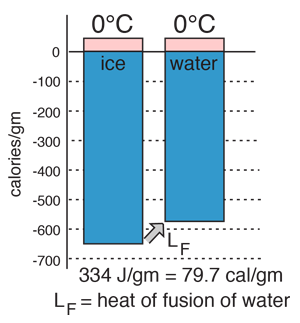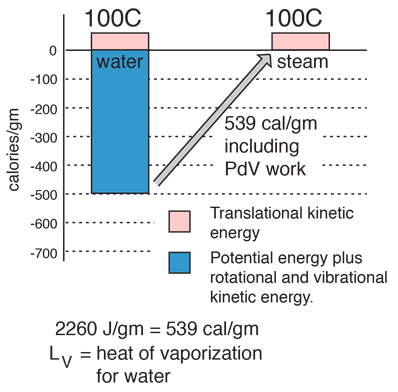# Heat of Fusion### The energy required to change a gram of a substance from the solid to the liquid state without changing its temperature is commonly called it's "heat of fusion". This energy breaks down the solid bonds, but leaves a significant amount of energy associated with the intermolecular forces of the liquid state.

 Water phase changes Table of heats of fusion Heat of fusion measurement
 Melting point depression by dissolved material
 Water
Index

Phase change concepts

 HyperPhysics***** Thermodynamics R Nave
Go Back

# Heat of Fusion Measurement

Measurement of the heat of fusion of water LF accurately requires that the ice to be melted is perfectly dry! The following shows that wet ice will yield a value below the standard value.where L*F is the experimental value obtained assuming that all the mass measured as mice is in fact ice. But if an incremental mass Δm is already water (wet ice), then the real value of the heat of fusion is obtained fromThe final expression for the experimental heat of fusion isThe experimental value L*F is seen to be too small since the denominator of the first expression for latent heat above contains the entire mass and is therefore too large. If 100 grams of water is started at 40 C and cooled to 10 C, an ice mass of 33.3 grams of perfectly dry ice would be required for a heat of fusion equal to 80 cal/gm. If one gram of the measured 33.3 gms were already melted, then the water would be cooled to 10.6 C and the experimental value for the heat of fusion would be 77.6 cal/gm.

 Water phase changes Heat of fusion Specific heat
 Water
Index

Phase change concepts

 HyperPhysics***** Thermodynamics R Nave
Go Back

# Heat of VaporizationThe energy required to change a gram of a liquid into the gaseous state at the boiling point is called the "heat of vaporization". This energy breaks down the intermolecular attractive forces, and also must provide the energy necessary to expand the gas (the PDV work). For an ideal gas , there is no longer any potential energy associated with intermolecular forces. So the internal energy is entirely in the molecular kinetic energy. The final energy is depicted here as being in translational kinetic energy, which is not strictly true. There is also some vibrational and rotational energy.

A significant feature of the vaporization phase change of water is the large change in volume that accompanies it. A mole of water is 18 grams, and at STP that mole would occupy 22.4 liters if vaporized into a gas. If the change is from water to steam at 100°C, rather than 0°C, then by the ideal gas law that volume is increased by the ratio of the absolute temperatures, 373K/273K, to 30.6 liters. Comparing that to the volume of the liquid water, the volume expands by a factor of 30600/18 = 1700 when vaporized into steam at 100°C. This is a physical fact that firefighters know, because the 1700-fold increase in volume when water is sprayed on a fire or hot surface can be explosive and dangerous.

One way to visualize this large volume change is to note the volume of 18 ml of water in a graduated cylinder as the volume occupied by Avogadro's number of water molecules in the liquid state. If converted into steam at 100°C this same mole of water molecules would fill a balloon 38.8 cm in diameter (15.3 inches).

 Water phase changes Table of heats of vaporization
 Some energy modeling calculations The heat of vaporization at body temperature is 580 cal/gm. Why?
 Water
Index

Phase change concepts

 HyperPhysics***** Thermodynamics R Nave
Go Back